# What mass of iron(III) oxide must be used to produce 64.28 g of iron?What is the maximum mass of aluminum oxide that could be produced along with 79.76 g iron?What mass of aluminum must be used to produce 80.05 g of iron?

Question
1 views

What mass of iron(III) oxide must be used to produce 64.28 g of iron?

What is the maximum mass of aluminum oxide that could be produced along with 79.76 g iron?

What mass of aluminum must be used to produce 80.05 g of iron?

check_circle

Step 1

The equation required to produce iron from iron(III)oxide is as follows,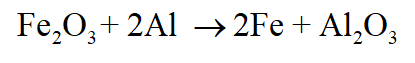Given grams of iron is converted into moles as follows,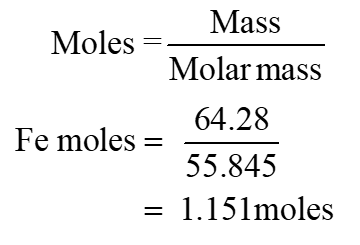Step 2

Observing the balanced equation shows that 2 moles of Fe is obtained from 1 mole of iron(III)oxide moles. Therefore, 1.151 moles of Fe is obtained from 0.5755 moles of Fe2O3.

Now, the moles of Fe2O3 is converted into mass as follows,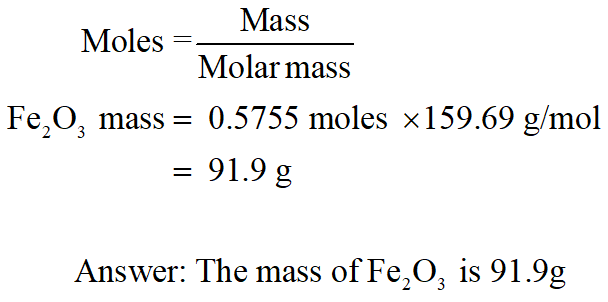Step 3

The equation required to produce iron from iron(III)oxide is as follows,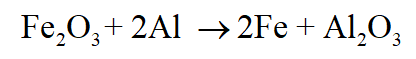Given grams of iron is converted into moles as follows,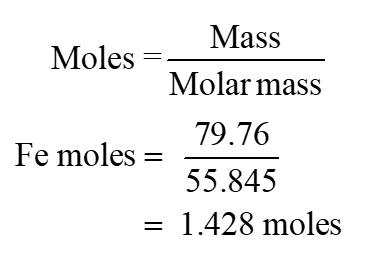Observing the balanced equation shows that 2 moles of Fe is produced along with 1 mole of Al2O3. Therefore, the mole of Al2O3 produced along with given grams of iron are 0.714 moles.

...

### Want to see the full answer?

See Solution

#### Want to see this answer and more?

Solutions are written by subject experts who are available 24/7. Questions are typically answered within 1 hour.*

See Solution
*Response times may vary by subject and question.
Tagged in

### Chemistry